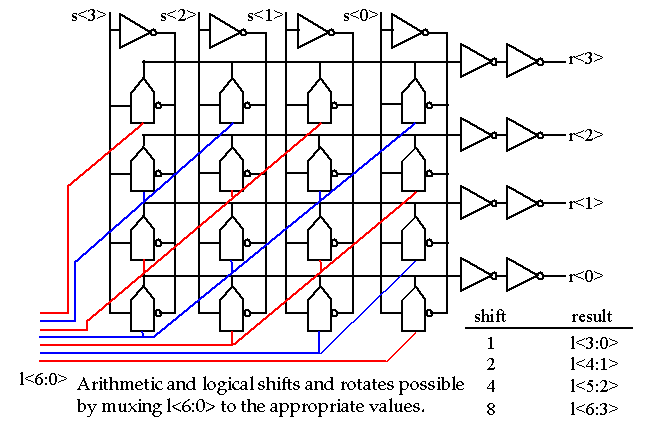## Digital Device Components

• A simple processor illustrates many of the basic components used in any digital system: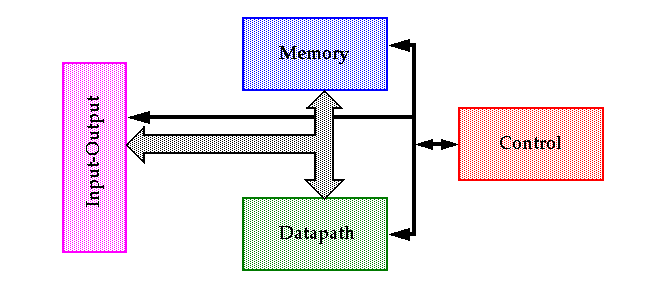• Datapath: The core -- all other components are support units that store either the results of the datapath or determine what happens in the next cycle.

## Digital Device Components

• Memory:
• A broad range of classes exist determined by the way data is accessed:
• Sequential vs. Random access
• Single-ported vs. Multi-ported access
• Or by their data retention characteristics:
• Dynamic vs. Static
• Stay tuned for a more extensive treatment of memories.

• Control:
• A FSM (sequential circuit) implemented using random logic, PLAs or memories.

• Interconnect and Input-Output:
• Parasitic resistance, capacitance and inductance affect performance of wires both on and off the chip.
• Growing die size increases the length of the on-chip interconnect, increasing the value of the parasitics.

## Digital Device Components

• Datapath elements include adders, multipliers, shifters, BFUs, etc.
• The speed of these elements often dominates the overall system performance so optimization techniques are important.

• However, as we will see, the task is non-trivial since there are multiple equivalent logic and circuit topologies to choose from, each with adv./disadv. in terms of speed, power and area.

• Also, optimizations focused at one design level, e.g., sizing transistors, leads to inferior designs.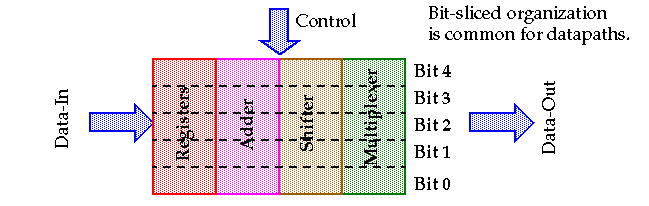• Let's start with addition, since it is very a common datapath element and often a speed-limiting element.

• Optimizations can be applied at the logic or circuit level.
• Logic-level optimization try to rearrange the Boolean equations to produce a faster or smaller circuit, e.g. carry look-ahead adder.
• Circuit-level optimizations manipulate transistor sizes and circuit topology to optimize speed.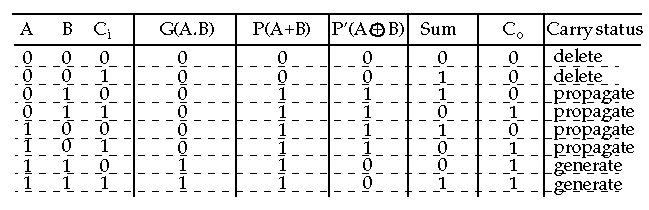• G(A.B) : (generate)
• Occurs when a Co is internally generated within the adder (occurs independent of Ci).

• P(A+B) : (propagate)
• Indicates that Ci is propagated (passed) to Co.

• P'(A XOR B) : (propagate)
• Used in some adders for the P term since it can be reused to generate the sum term.

• D( A . B ) : (delete)
• Ensures that a carry bit will be deleted at C o .

• The Boolean expressions for S and C o are:
• Sum = A.B.C i + A.B.C i + A.B.C i + A.B.C i = A XOR B XOR C
• Carry = A.B + A.C i + B.C i

• But S and C o can be written in terms of G and P':
• C o (G, P') = G + P'C i (or P in this case).
• S(G, P') = P' XOR C i

• Note that G and P' are INdependent upon C i .
• (Also, C o and S can be expressed in terms of delete (D)).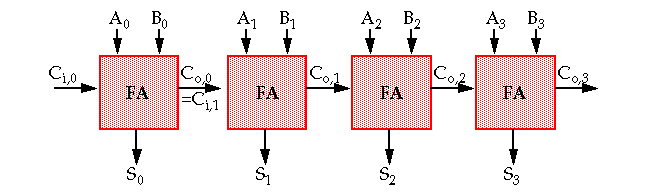• The critical path (worst case delay over all possible inputs) is a ripple from lsb to msb .

• The delay in this case is proportional to the number of bits, N, in the input words:
• t adder = (N - 1)t carry + t sum
• where t carry and t sum equal the propagation delays from C i to C o & S.
• One possible worst case bit pattern (from lsb to msb ) is:
• A: 00000001; B: 01111111
• Convince yourself that this is true.

• Note that when optimizing this structure, it is far more important to optimize t carry than t sum .

• The inverting property of a full adder can be used to achieve this goal: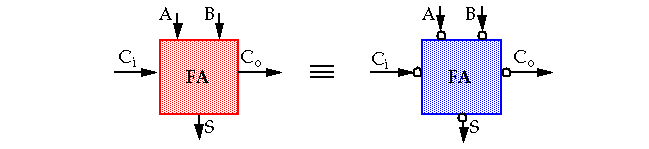• Thus,
• S(A, B, Ci) = S(A, B, Ci)
• Co(A, B, Ci) = Co(A, B, Ci)

• One possible (un-optimized) implementation:• C o is reused in the S term as:
• Sum = A.B.Ci + (A + B + Ci)Co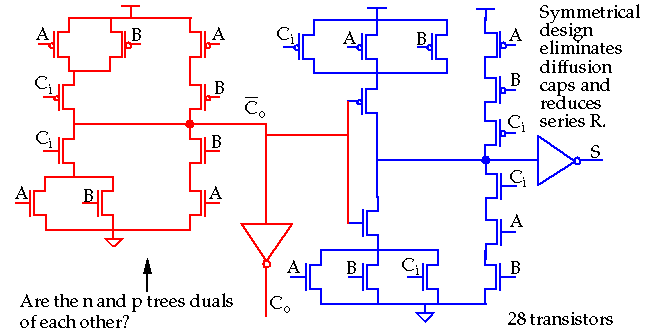• Even with some design tricks, e.g., transistors on the critical path, C i , placed closest to the output and symmetrical design, this implementation is slow.

• The load capacitance in previous version on C o consists of 2 diffusion capacitances (inverter) and 6 (next bit) gate capacitances: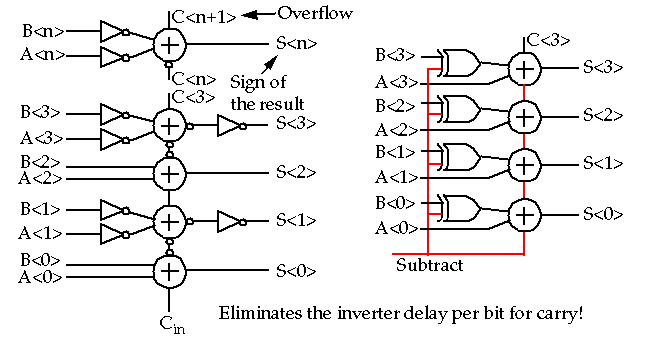• This version increases Co's load to 4 diffusion caps, 2 internal (sum) gate caps plus the 6 (next bit) gate caps.

• Serial addition can be used if area is a concern: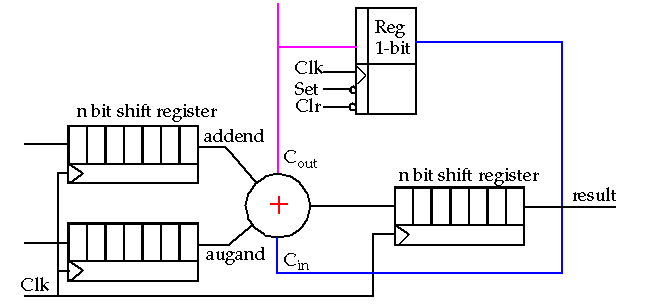• In this case, you want equal Sum and Carry delays in order to minimize clock cycle time.

• Bit-level pipelining can be used to break the dependency between addition time and the number of bits by inserting FAs between each register bit.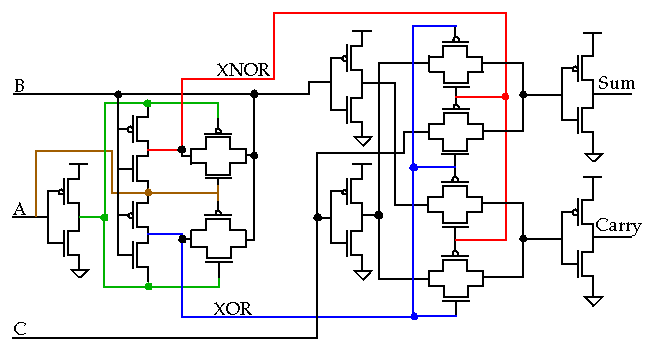• Total transistors is 26! Can reduce to 24 by using an inverter for XNOR (see Weste and Eshraghian for an 18 transistor implementation).
• Note: Sum and Carry delay times are approximately equal.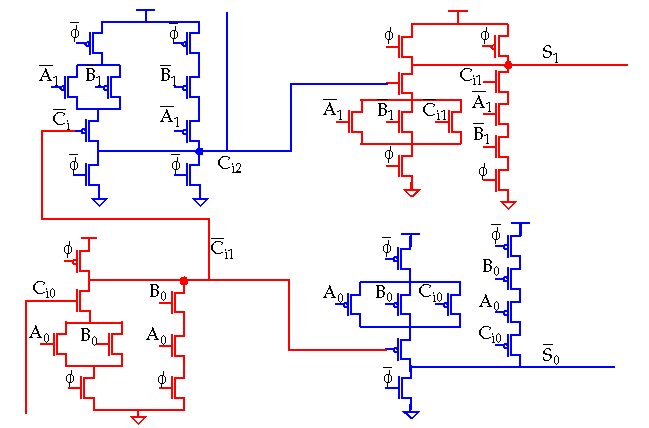• A chain of pass-transistors are used to implement the carry chain.• Precharge: All intermediate nodes, e.g. Co,0, charged to VDD.
• Evaluate: Node Co,k is discharged if there is an incoming carry, Ci,0 and the previous propagate signals are high, P0 to Pk-1.

• Only 4 diffusion capacitances are present per node but the distributed RC-nature of the chain results in delay that is quadratic with number of bits.
• Buffers and/or transistor sizing can be used to improve performance.

• Consider the worst case delay of the carry chain: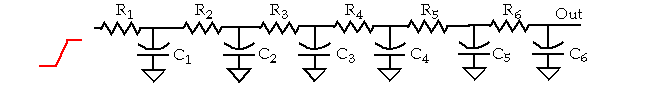• Elmore delay is given by: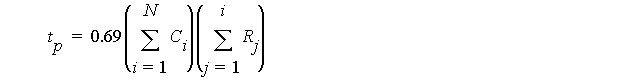• The delay of the RC network is then:
• t p = 0.69(C 1 R 1 + C 2 (R 1 + R 2 ) + C 3 (R 1 + R 2 + R 3 ) + C 4 (R 1 + R 2 + R 3 + R 4 ) +
• C 5 (R 1 + R 2 + R 3 + R 4 + R 5 ) + C 6 (R 1 + R 2 + R 3 + R 4 + R 5 + R 6 )
• Since R 6 appears 6 times in the expression, it makes sense to minimize its contribution.

• Note that reducing R by a factor, e.g. k , at each stage increases the capacitance by a factor k and increases area.
• A k-factor of 1.5, reduces delay by 40% and increases area by 3.5X.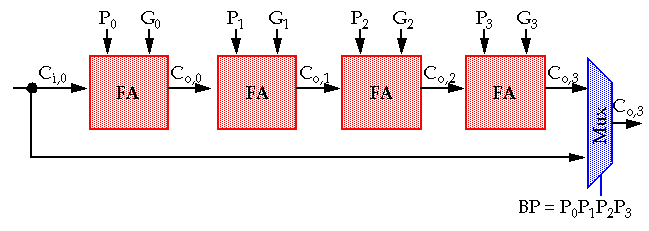• Assume A k and B k (for k = 1...3) are set such that all P k (propagate) are high.
• In this case, an incoming carry C i,0 = 1, propagates along the complete chain and C o,3 = 1.
• In other words:
• if (P 0 P 1 P 2 P 3 == 1) then C o,3 = C i,0 else either DELETE or GENERATE occurred.

• One way around waiting for the incoming carry is to compute the result of both possible values in advance and let the incoming carry select the correct result.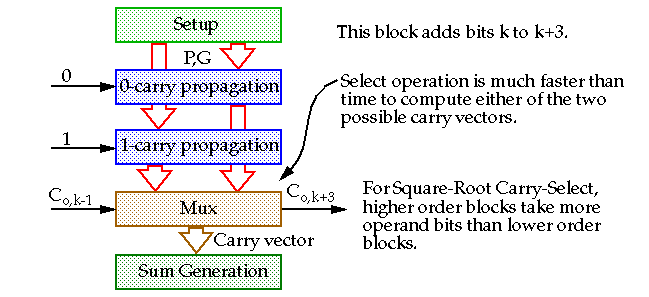• A Square-Root Carry-Select Adder (delay = O(N 1/2 )) is constructed by increasing the number of input bits in each block from lsb to msb .

• Compute the carries to each stage in parallel.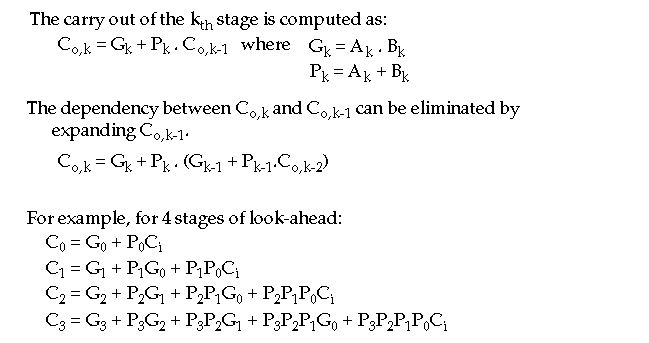• Note that the low-order terms, e.g., P 0 and G 0 , appear in the expression for every bit, making the fanout load large.

• One possible implementation without using simple logic gates.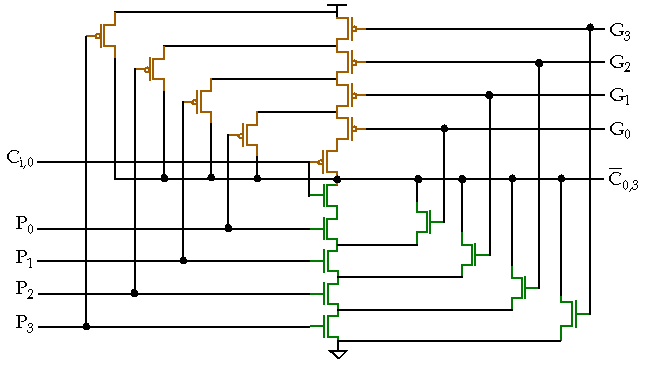• Size and fan-in of the gates limit the size to about four.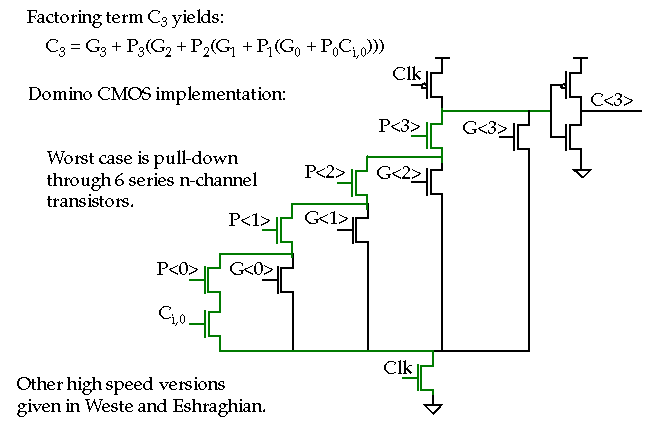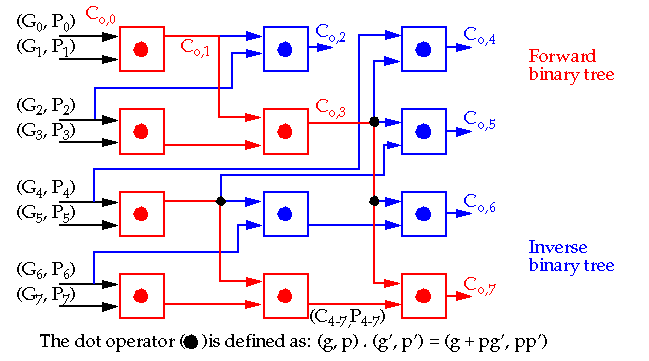• The number of logic levels is proportional to log 2 N, fan-in is limited and the layout is compact (jigsaw puzzle) (see Rabaey for details).

## Datapath Operators: Comparison

• Magnitude Comparators :
• May be built from an adder, complementer (XOR gates) and a zero detect unit.• Think about the modifications necessary to make it a signed comparator (Hint: A couple of XOR gates).

## Datapath Operators: Binary Counters

• Asynchronous: Based on the Toggle register.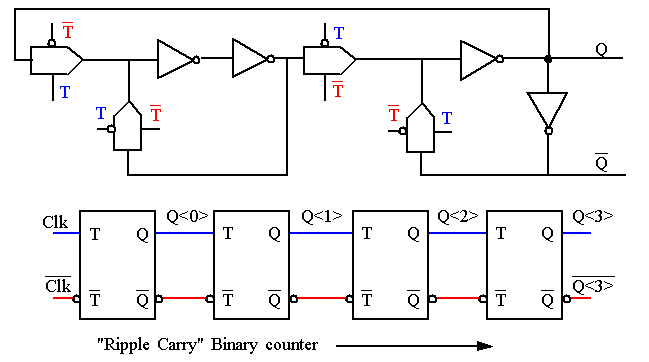• Not a good choice for performance and testability (with no reset).

## Datapath Operators: Binary Counters

• Synchronous counter.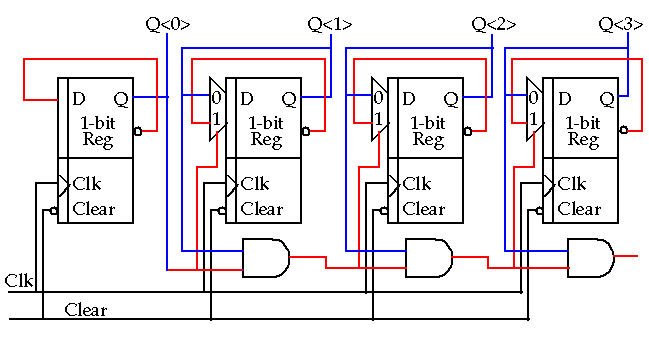• Replace AND gate with an adder for up/down counting capability.
• Weste and Eshraghian also show a version that can be initialized.

## Datapath Operators: Multiplication

• Multiplication can be broken down into two steps:
• Computation of partial products.
• Accumulation of the shifted partial products.• Multipliers may be classified by the format in which data words are accessed:
• Serial
• Serial/parallel
• Parallel

• The parallel form computes the partial products in parallel.

## Datapath Operators: Multiplication

• Parallel Unsigned Multiplication: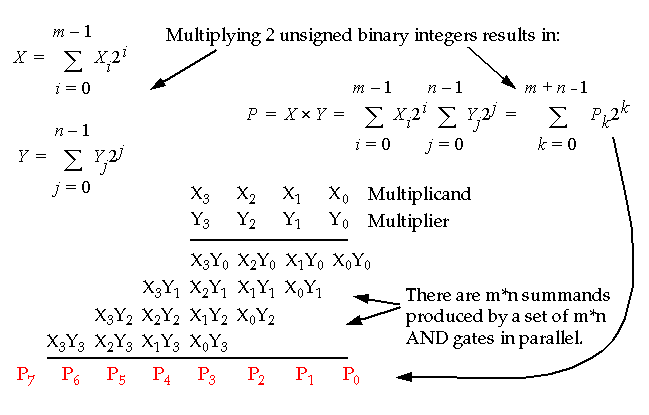## Datapath Operators: Multiplication

• Parallel Multiplication: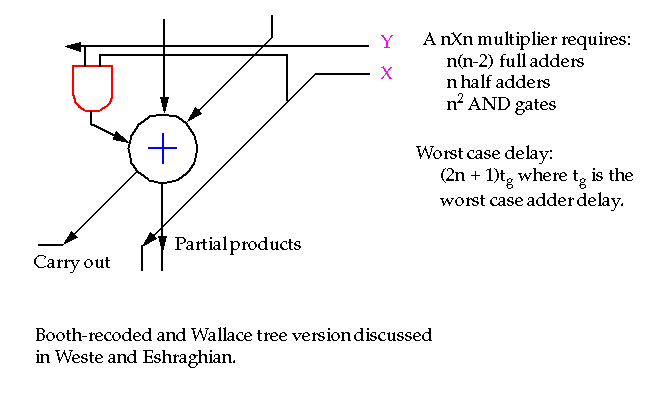## Datapath Operators: Multiplication

• Parallel Signed Multiplication: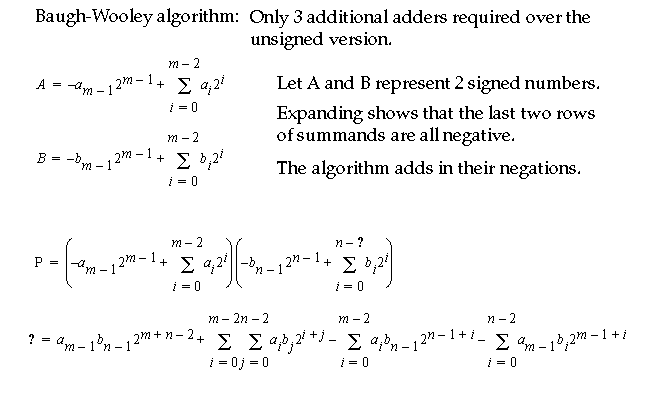## Datapath Operators: Multiplication

• Parallel Signed Multiplication: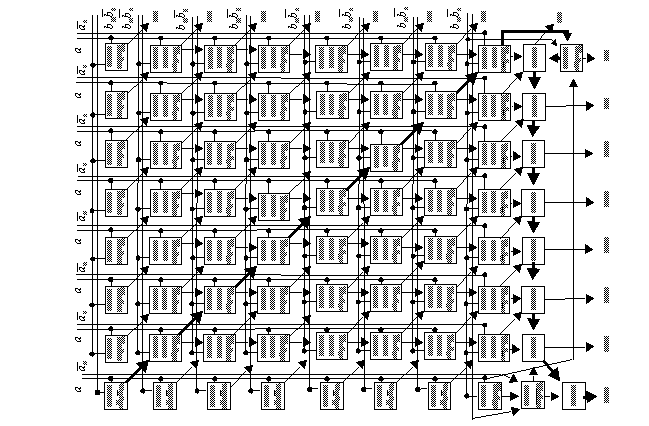## Datapath Operators: Multiplication

• Serial Unsigned Multiplication: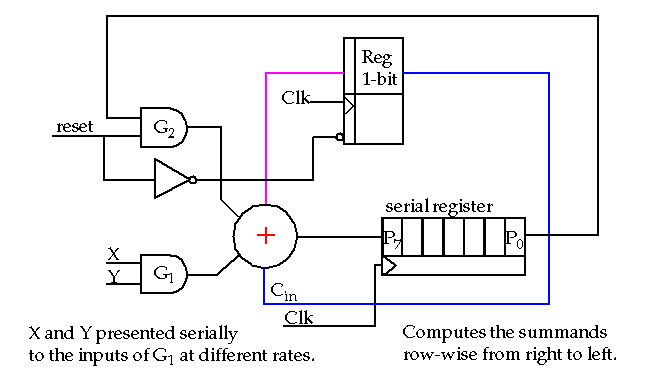• Serial/Parallel Unsigned Multiplier shown in Weste and Eshraghian.

## Datapath Operators: Shifters

• Right/Left 1-bit shifter: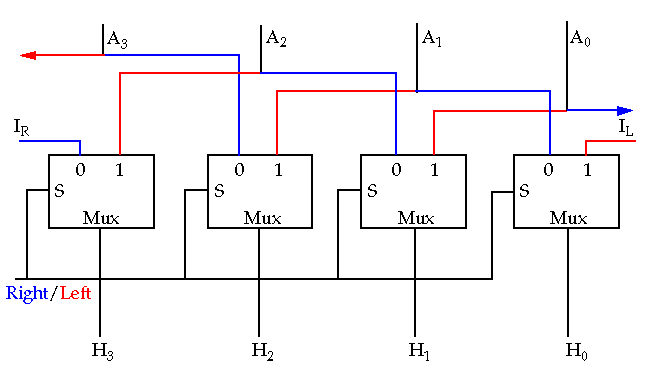## Datapath Operators: Shifters

• Barrel shifter: